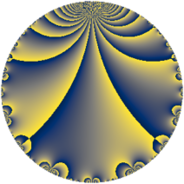# Properties

 Label 16.4.aLevel $16$ Weight $4$ Character orbit 16.a Rep. character $\chi_{16}(1,\cdot)$ Character field $\Q$ Dimension $1$ Newform subspaces $1$ Sturm bound $8$ Trace bound $0$

# Related objects

## Defining parameters

 Level: $$N$$ $$=$$ $$16 = 2^{4}$$ Weight: $$k$$ $$=$$ $$4$$ Character orbit: $$[\chi]$$ $$=$$ 16.a (trivial) Character field: $$\Q$$ Newform subspaces: $$1$$ Sturm bound: $$8$$ Trace bound: $$0$$

## Dimensions

The following table gives the dimensions of various subspaces of $$M_{4}(\Gamma_0(16))$$.

Total New Old
Modular forms 9 2 7
Cusp forms 3 1 2
Eisenstein series 6 1 5

The following table gives the dimensions of the cuspidal new subspaces with specified eigenvalues for the Atkin-Lehner operators and the Fricke involution.

$$2$$Dim
$$+$$$$1$$

## Trace form

 $$q + 4 q^{3} - 2 q^{5} - 24 q^{7} - 11 q^{9} + O(q^{10})$$ $$q + 4 q^{3} - 2 q^{5} - 24 q^{7} - 11 q^{9} + 44 q^{11} + 22 q^{13} - 8 q^{15} + 50 q^{17} - 44 q^{19} - 96 q^{21} + 56 q^{23} - 121 q^{25} - 152 q^{27} + 198 q^{29} + 160 q^{31} + 176 q^{33} + 48 q^{35} - 162 q^{37} + 88 q^{39} - 198 q^{41} - 52 q^{43} + 22 q^{45} - 528 q^{47} + 233 q^{49} + 200 q^{51} - 242 q^{53} - 88 q^{55} - 176 q^{57} + 668 q^{59} + 550 q^{61} + 264 q^{63} - 44 q^{65} - 188 q^{67} + 224 q^{69} - 728 q^{71} + 154 q^{73} - 484 q^{75} - 1056 q^{77} + 656 q^{79} - 311 q^{81} - 236 q^{83} - 100 q^{85} + 792 q^{87} + 714 q^{89} - 528 q^{91} + 640 q^{93} + 88 q^{95} - 478 q^{97} - 484 q^{99} + O(q^{100})$$

## Decomposition of $$S_{4}^{\mathrm{new}}(\Gamma_0(16))$$ into newform subspaces

Label Dim $A$ Field CM Traces A-L signs $q$-expansion
$a_{2}$ $a_{3}$ $a_{5}$ $a_{7}$ 2
16.4.a.a $1$ $0.944$ $$\Q$$ None $$0$$ $$4$$ $$-2$$ $$-24$$ $+$ $$q+4q^{3}-2q^{5}-24q^{7}-11q^{9}+44q^{11}+\cdots$$

## Decomposition of $$S_{4}^{\mathrm{old}}(\Gamma_0(16))$$ into lower level spaces

$$S_{4}^{\mathrm{old}}(\Gamma_0(16)) \cong$$ $$S_{4}^{\mathrm{new}}(\Gamma_0(8))$$$$^{\oplus 2}$$